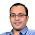## Dec 9, 2013

### Picking K elements randomly

Problem 1: Consider the problem of picking K elements randomly from N elements. Suggest an algorithm. What is the time and space complexity of your algorithm?

Problem 2: Now consider that the stream is infinitely long (i.e. N is unknown). Now how do we pick K elements randomly.

Update (24 June 2014):
Solution: Posted by Himanshu (Prob 1), PlacementIITB2014 (Prob2) and Sid (Prob1 and Prob2) in comments! Thanks

claimtoken-52ac74fbddf1e

#### 6 comments:

1.for problem 1:
first we pick one element randomly from N elements , then another one from remaining N-1 elements..so on..till we get K elements;
One way to code this is..after getting the first element we can swap this element with first element in an array. Now we need to find 2nd random element from a to a[N-1]. Next we swap a & random element.
After the K steps our initial array contains random elements (from a to a[k-1]). We don't need any extra space.
Time complexity: we are just swapping the random elements. Picking a random element is O(1) (not sure).

1.Thanks Himanshu. This is Fisher Yattes Shuffle. Thanks - Wiki Link: http://en.wikipedia.org/wiki/Fisher-Yates_shuffle

2.Problem 1: Generate a random number i between 1 and N. Select the ith element, and swap the Nth element with the ith in the array. Now, generate a random number j between 1 and N-1. Select the jth element, and swap the (N-1)th element with the jth in the array. Continue until K. (This is similar to Fisher Yates shuffle)

Problem 2: Select the ith element in the stream with probability min(1, K/i). If more than K elements have already been selected, replace any of the existing elements at random. (This is also called Reservoir Sampling)

1.Thanks Sid. Both solutions are bang on! Thanks

3.I think Reservoir Algorithm will work - http://en.wikipedia.org/wiki/Reservoir_sampling

1.For the second part, Reservoir Sampling would work. Thanks

### Fraction Brainteaser

Source: Sent to me by Gaurav Sinha Problem: Siddhant writes a Maths test and correctly answers 5 out of 6 Arithmetic questions and 20...